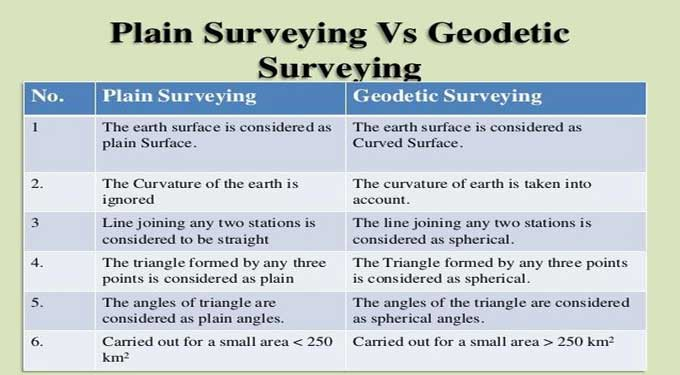# Plain Surveying versus Geodetic Surveying: What's the difference?The civil surveying operation involves assessing and recording details about a particular parcel of land. Construction projects can then be planned based on these observations.

To determine the three-dimensional relationship between various locations, civil engineers use surveying. To design a construction plan or map, surveyors analyze and record the features of a land area span.

### Define Plain Surveying

In plane surveying, the surface of the earth is treated as a plane surface, without taking into account its curvature.

During plane surveying, the earth's surface is treated as a plane surface. It is used for small projects covering an area less than 250 square kilometers. The curvature of the earth is not taken into account when calculating distances.

1. Earth's plain surfaces are considered to be those on the surface.
2. There is no consideration for the curvature of the earth.
3. Three points forming a triangle are considered plain.
4. A small area of 250 km was covered in this study.
5. Instruments used in plane surveying include chains, measuring tapes, theodolites, etc.
6. There is a low degree of survey accuracy.
7. Any line connecting two stations is considered straight.

### Define Geodetic Surveying

Using geodetic principles, geodetic surveying takes into account the curvature of the earth's surface in order to achieve high precision.

Geodetic surveying must be used if the area to be surveyed is greater than 1000 km2, since angles measured on the earth's surface cannot be on a plane surface but on a curved surface.

When measuring the surface of the earth, geodetic surveying takes into account the curvature of the earth. It provides control points to which smaller surveys can be connected when the survey covers a large area more than 250 square kilometers and accuracy is great. Geodetic survey is commonly used when surveys span over larger areas more than 250 square kilometers.

1. It is considered that the earth surface has a curved shape since it is curved.
2. We take into account the curvature of the earth.
3. Spherical triangles are formed by any three points.
4. Applied to an area of more than 250 km2.
5. GPS and more precise instruments are used in geodetic surveying.
6. There is high accuracy in the survey.
7. Any line connecting two stations is considered spherical.

### Difference between Plain Surveying & Geodetic Surveying

The main difference between geodetic and plane surveying is in the assumptions used to compute the results.

The plane surveying method presumes that the earth is flat when it is conducted. In plane surveying calculations, the curvature or spherical shape of the earth is not taken into account. A geodetic survey considers the curvature or spherical shape of the earth when surveying.

The curved shape of the earth is taken into account in geodetic surveying or geodesy. It is usually undertaken by government agencies and is rational in nature as an example, the United States Government's National Geological Survey.

Individuals can determine whether their objective is scientific or artistic, i.e., determining the size and shape of the earth or creating accurate maps of large areas.

Instruments and methods are used to determine the absolute positions of points on the surface of the earth.

These points are used as control points in surveys covering smaller areas. The mean spherical shape of the earth must be used when calculating large areas.

In areas such as a province or a city, assuming the earth is a perfect sphere simplifies the analysis significantly.

To learn more, watch the following video tutorial.

Video Source: Civil Snapshot

Anyhow, it is necessary to know spherical trigonometry and calculus. Geodetic computations now commonly use three-dimensional, earth-centered, Cartesian coordinate systems.